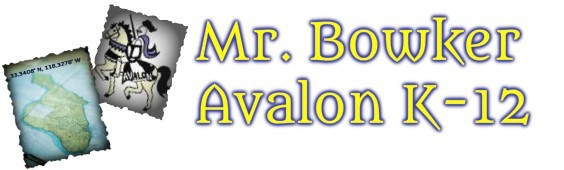Circumference, Perimeter and Area
4 years ago
jbowker88
Save
Edit
Host a game
Live GameLive
Homework
Solo Practice
Practice18 QuestionsShow answers
• Question 1
180 secondsQ. What is the diameter?
5 in
10 in
3.14 in
15.84 in
• Question 2
120 secondsQ. What is the circumference?
48
96
24
18
• Question 3
120 secondsQ. Find the area of the circle
43.96
153.86
87.82
307.72
• Question 4
120 secondsQ. Does this picture show the diameter or radius of a circle?
Diameter
• Question 5
30 secondsQ. Does this picture show the diameter or radius of a circle?
Diameter
• Question 6
60 secondsQ. Find the area of the circle
56.52
254.34
1017.36
113.04
• Question 7
60 secondsQ. Find the circumference of the circle.
113.04
1017.36
254.34
56.52
• Question 8
30 secondsQ. What is the diameter of this circle?
3.5
3
5
7
• Question 9
30 secondsQ. What is the radius of this circle?
18
10
9
8
• Question 10
180 secondsQ. Find the perimeter of the figure.  Use 3.14 for pi.
20.56 in.
17.3 in.
17.8 in.
31.88 in.
• Question 11
180 secondsQ. Find the perimeter of the figure.
10 ft
14 ft
20 ft
18 ft
• Question 12
180 secondsQ. Find the perimeter of the figure.
86 yd.
32 yd.
33 yd.
53 yd.
• Question 13
180 secondsQ. Find the perimeter of the figure.
33 km.
36 km.
32 km.
33 km.
• Question 14
180 secondsQ. Find the perimeter of the figure.
32 mi
33 mi
36 mi
35 mi
• Question 15
300 secondsQ. perimeter
81 km
85 km
91 km
95 km
• Question 16
300 secondsQ. area?
16.5 u sq
25 u sq
20 u sq
34 u sq
• Question 17
300 secondsQ. perimeter?
24 cm
27 cm
30 cm
33 cm
• Question 18
300 secondsQ. perimeter